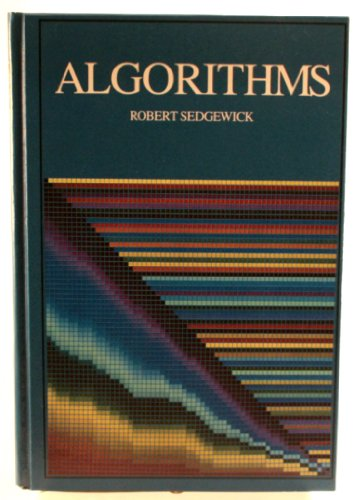## bean bag win

Posted in Books, Kids, pictures, R with tags , , , , on May 19, 2021 by xi'anA quick riddle from The Riddler, where a multiple step game sees a probability of a 3 point increase of .4 and a probability of a 1 point increase of .3 with a first strategy (A), versus a probability of a 3 point increase of .4 and a probability of a 1 point increase of .3 with a second strategy (B), and a sure miss third strategy (C). The goal is to optimise the probability of hitting exactly 3 points after 4 steps.

The optimal strategy is to follow A while the score is zero, C when the score is 3, and B otherwise. The corresponding winning probability is 0.8548, as checked by the following code

win=function(n=1,s=0){
if(n==4)return((s==3)+.4*(!s)+.8*(s==2))
else{return(max(c(
.4*win(n+1,s+3)+.3*win(n+1,s+1)+.3*win(n+1,s),
.1*win(n+1,s+3)+.8*win(n+1,s+1)+.1*win(n+1,s),
win(n+1,s))))}}


## poems that solve puzzles [book review]

Posted in Books, Kids, University life with tags , , , , , , , , , , , , , , , , , , on January 7, 2021 by xi'anUpon request, I received this book from Oxford University Press for review. Poems that Solve Puzzles is a nice title and its cover is quite to my linking (for once!). The author is Chris Bleakley, Head of the School of Computer Science at UCD.

“This book is for people that know algorithms are important, but have no idea what they are.”These is the first sentence of the book and hence I am clearly falling outside the intended audience. When I asked OUP for a review copy, I was more thinking in terms of Robert Sedgewick’s Algorithms, whose first edition still sits on my shelves and which I read from first to last page when it appeared [and was part of my wife’s booklist]. This was (and is) indeed a fantastic book to learn how to build and optimise algorithms and I gain a lot from it (despite remaining a poor programmer!).

Back to poems, this one reads much more like an history of computer science for newbies than a deep entry into the “science of algorithms”, with imho too little on the algorithms themselves and their connections with computer languages and too much emphasis on the pomp and circumstances of computer science (like so-and-so got the ACM A.M. Turing Award in 19… and  retired in 19…). Beside the antique algorithms for finding primes, approximating π, and computing the (fast) Fourier transform (incl. John Tukey), the story moves quickly to the difference engine of Charles Babbage and Ada Lovelace, then to Turing’s machine, and artificial intelligence with the first checkers codes, which already included some learning aspects. Some sections on the ENIAC, John von Neumann and Stan Ulam, with the invention of Monte Carlo methods (but no word on MCMC). A bit of complexity theory (P versus NP) and then Internet, Amazon, Google, Facebook, Netflix… Finishing with neural networks (then and now), the unavoidable AlphaGo, and the incoming cryptocurrencies and quantum computers. All this makes for pleasant (if unsurprising) reading and could possibly captivate a young reader for whom computers are more than a gaming console or a more senior reader who so far stayed wary and away of computers. But I would have enjoyed much more a low-tech discussion on the construction, validation and optimisation of algorithms, namely a much soft(ware) version, as it would have made it much more distinct from the existing offer on the history of computer science.

[Disclaimer about potential self-plagiarism: this post or an edited version of it will eventually appear in my Books Review section in CHANCE.]

## not a Bernoulli factory

Posted in Books, Kids, pictures, R with tags , , , , , , , on May 20, 2020 by xi'an

A Riddler riddle I possibly misunderstood:

Four isolated persons are given four fair coins, which can be either flipped once or returned without being flipped. If all flipped coins come up heads, the team wins! Else, if any comes up tails, or if no flip at all is done, it looses. Each person is further given an independent U(0,1) realisation. What is the best strategy?

Since the players are separated, I would presume the same procedure is used by all. Meaning that a coin is tossed with probability p, ie if the uniform is less than p, and untouched otherwise. The probability of winning is then

4(1-p)³p½+6(1-p)³p½²+4(1-p)p³½³+p⁴½⁴

which is maximum for p=0.3420391, with a winning probability of 0.2848424.And an extra puzzle for free:

solve $$x⌊x⌊x⌊x⌋⌋⌋=2020$$

Where the integral part is the integer immediately below x. Puzzle that I first fail solving by brute force, because I did not look at negative x’s… Since the fourth root of 2020 is between 6 and 7, the solution is either x=6+ε or x=-7+ε, with ε in (0,1). The puzzle then becomes either

$$(6+ε)⌊(6+ε)⌊(6+ε)⌊6+ε⌋⌋⌋ = (6+ε)⌊(6+ε)⌊36+6ε⌋⌋ = (6+ε)⌊(6+ε)(36+⌊6ε⌋)⌋ = 2020$$

where there are 6 possible integer values for $$⌊6ε⌋, with only ⌊6ε⌋=5 being possible, turning the equation into$$

$$(6+ε)⌊41(6+ε)⌋ = (6+ε)(246+⌊41ε⌋) = 2020$$

where again only $$⌊42ε⌋$$=40 being possible, ending up with

$$1716+286ε = 2020$$

which has no solution in (0,1). In the second case

(-7+$$ε$$)$$⌊(-7+ε)⌊(-7+ε)⌊-7+ε⌋⌋⌋$$ = (-7+$$ε$$)$$⌊(-7+ε)(49+⌊-7ε⌋)⌋ = 2020$$

shows that only $$⌊-7ε⌋$$=-3 is possible, leading to

(-7+$$ε$$)$$⌊46(-7+ε))⌋$$ = (-7+$$ε$$) ($$-322+⌊46ε⌋)=2020$$

with only $$⌊46ε⌋$$=17 possible, hence

2135-305$$ε$$=2020

and

$$ε$$=115/305.

A brute force simulated annealing resolution returns x=-6.622706 after 10⁸ iterations. A more interesting question is to figure out the discontinuity points of the function

$$ℵ(x) = x$$$$⌊x⌊x⌊x⌋⌋⌋$$

as they seem to be numerous:For instance, only 854 of the first 2020 integers enjoy a solution to $$ℵ(x)$$=n.

## R puzzle

Posted in Statistics with tags , , on September 12, 2019 by xi'an

Can you guess the meaning of the following R code

"?"=u\164f8ToI\x6Et;'!'=prod;!{
y<-xtabs(~?readLines())}%in%{
z<-y}&z>T##&[]>~48bEfILpu

If not (!), the explanation is provided in Robin’s answer to a codegolf puzzle.

## who’s that travelling salesman path?!

Posted in Statistics with tags , , , on July 18, 2017 by xi'an